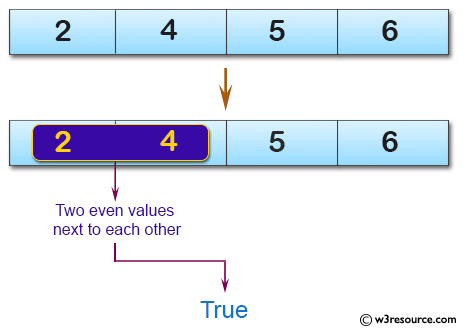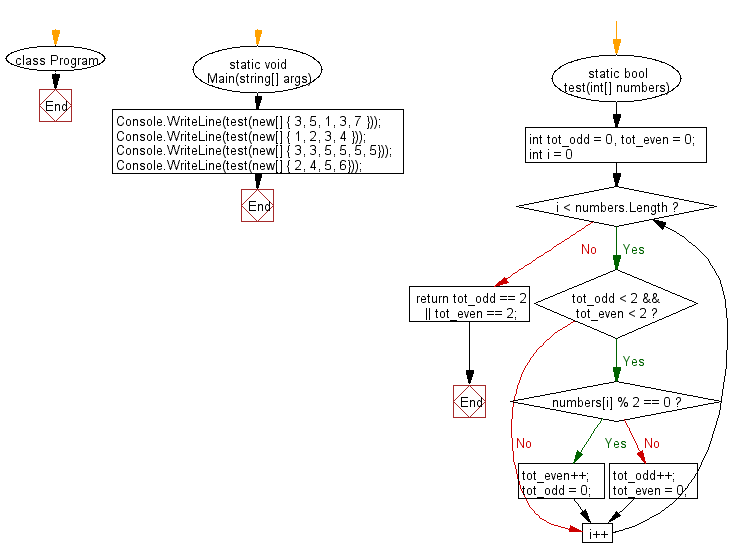﻿ C# - Check if an array has 2 even or odd next to each other# C# Sharp Basic Algorithm Exercises: Check a given array of integers and return true if the given array contains either 2 even or 2 odd values all next to each other

## C# Sharp Basic Algorithm: Exercise-122 with Solution

Write a C# Sharp program to check a given array of integers and return true if the given array contains either 2 even or 2 odd values all next to each other.

Pictorial Presentation:Sample Solution:

C# Sharp Code:

``````using System;
namespace exercises
{
class Program
{
static void Main(string[] args)
{
Console.WriteLine(test(new[] { 3, 5, 1, 3, 7 }));
Console.WriteLine(test(new[] { 1, 2, 3, 4 }));
Console.WriteLine(test(new[] { 3, 3, 5, 5, 5, 5}));
Console.WriteLine(test(new[] { 2, 4, 5, 6}));
}
static bool test(int[] numbers)
{
int tot_odd = 0, tot_even = 0;

for (int i = 0; i < numbers.Length; i++)
{
if (tot_odd < 2 && tot_even < 2)
{
if (numbers[i] % 2 == 0)
{
tot_even++;
tot_odd = 0;
}
else
{
tot_odd++;
tot_even = 0;
}
}
}
}
}
}
```
```

Sample Output:

```True
False
True
True
```

Flowchart:C# Sharp Code Editor:

Improve this sample solution and post your code through Disqus

What is the difficulty level of this exercise?

Test your Programming skills with w3resource's quiz.

﻿